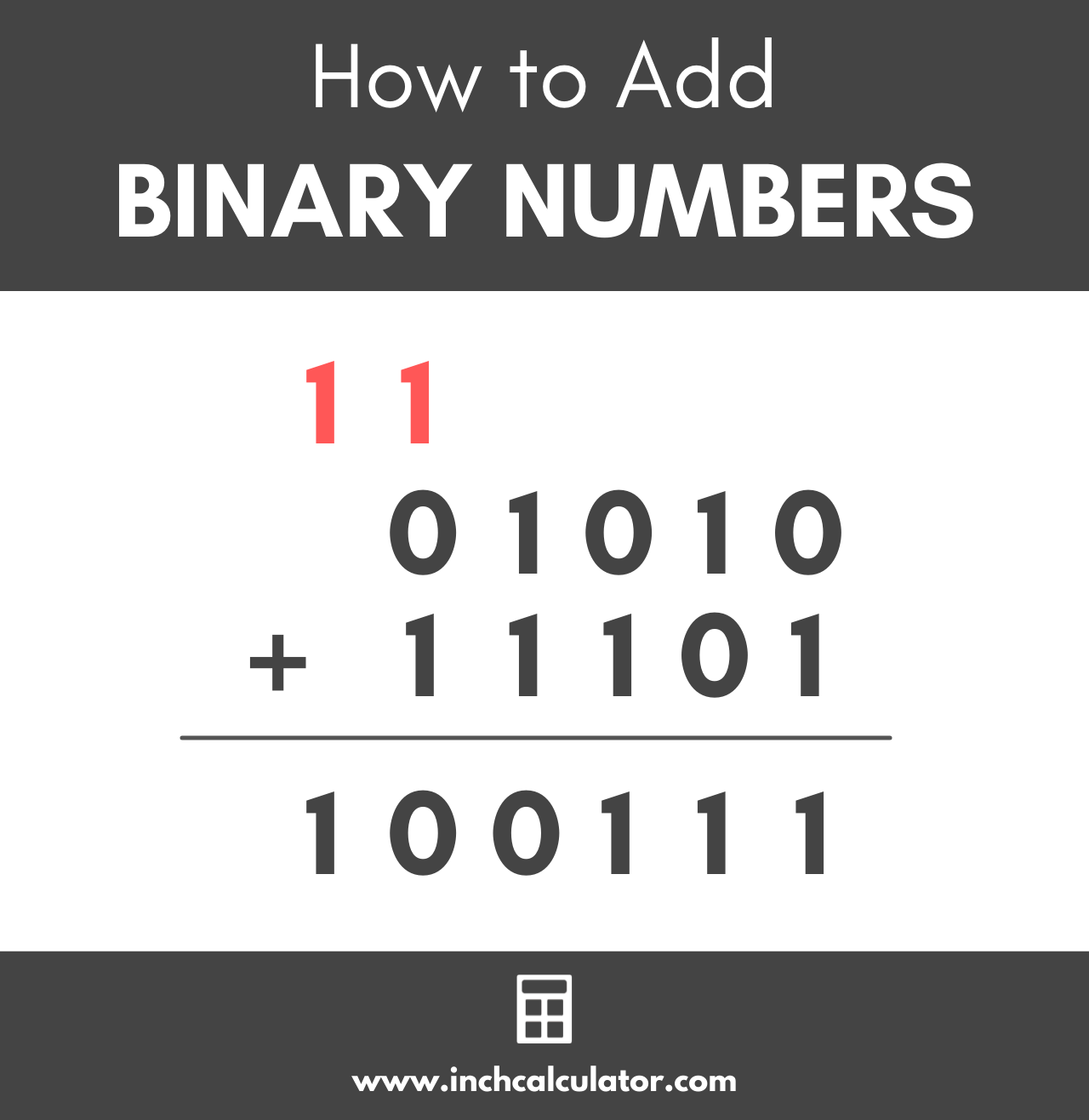# Binary Calculator

Add, subtract, multiply, or divide two binary, hex, or decimal numbers using the binary calculator below.

## How to Add Binary Numbers

Unlike the decimal system, the binary system is a base 2 number system and only uses the digits 0 and 1.

Adding binary numbers is very similar to adding decimal numbers, and you can do it using the place value.So, to add two binary numbers, write the second number below the first number, aligning the right digits, like this:

 0 1 0 1 0 + 1 1 1 0 1

Starting at the right-most number, add the top and bottom values together. In this case, 0 + 1 is equal to 1.

 0 1 0 1 0 + 1 1 1 0 1 1

Then, continue with the second number from the right; 1 + 0 is equal to 1.

 0 1 0 1 0 + 1 1 1 0 1 1 1

Move on to the third number from the right; 0 + 1 is equal to 1 again.

 0 1 0 1 0 + 1 1 1 0 1 1 1 1

With the next column, things start to get interesting. Adding 1 + 1 is equal to 2 in the decimal system, but the binary system only uses the digits 1 and 0.

In binary, 1 + 1 is equal to 10. In our problem, carry the leading 1 to the top of the problem over the first column of digits and move the trailing 0 to the bottom.

 1 0 1 0 1 0 + 1 1 1 0 1 0 1 1 1

In the next step, add the 1 at the top of the first column with the other values; 1 + 0 + 1 is equal to 10 in binary. You’ll need to carry to 1 to the top again and the 0 to the bottom like this:

 1 1 0 1 0 1 0 + 1 1 1 0 1 0 0 1 1 1

The final step is to move the final 1 from the very top to the bottom since 1 + 0 + 0 is equal to 1. Thus the answer is 100111.

 1 1 0 1 0 1 0 + 1 1 1 0 1 1 0 0 1 1 1